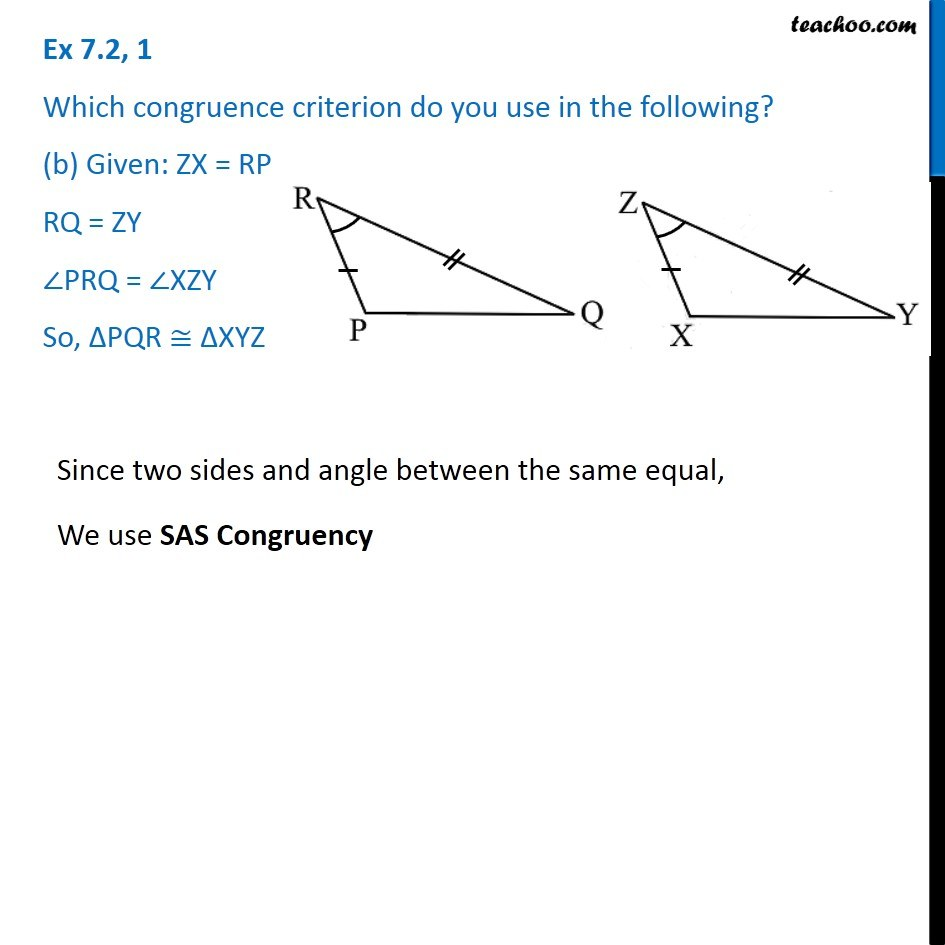1. Chapter 7 Class 7 Congruence of Triangles
2. Serial order wise
3. Ex 7.2

Transcript

Ex 7.2, 1 Which congruence criterion do you use in the following? (b) Given: ZX = RP RQ = ZY ∠PRQ = ∠XZY So, ∆PQR ≅ ∆XYZ Since two sides and angle between the same equal, We use SAS Congruency